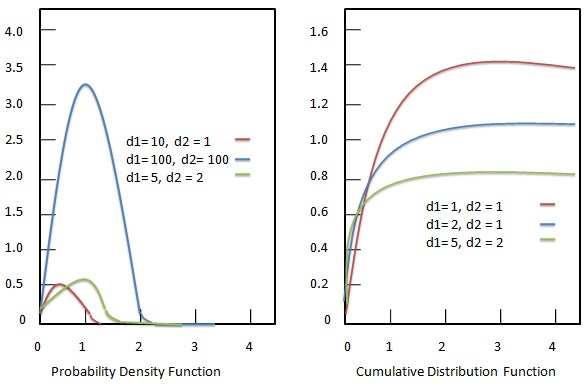# Statistics - F distribution

The F distribution (Snedecor's F distribution or the Fisher�Snedecor distribution) represents continuous probability distribution which occurs frequently as null distribution of test statistics. It happens mostly during analysis of variance or F-test.### Probability density function

Probability density function of F distribution is given as:

## Formula

${ f(x; d_1, d_2) = \frac{\sqrt{\frac{(d_1 x)^{d_1} d_2^{d_2}}{(d_1x+d_2)^{d_1+d_2}}}}{x \beta (\frac{d_1}{2}, \frac{d_2}{2})} }$

Where −

• ${d_1}$ = positive parameter.

• ${d_2}$ = positive parameter.

• ${x}$ = random variable.

### Cumulative distribution function

Cumulative distribution function of F distribution is given as:

## Formula

${ F(x; d_1, d_2) = I_{\frac{d_1x}{d_1x+d_2}}(\frac{d_1}{2}, \frac{d_2}{2})}$

Where −

• ${d_1}$ = positive parameter.

• ${d_2}$ = positive parameter.

• ${x}$ = random variable.

• ${I}$ = lower incomplete beta function.